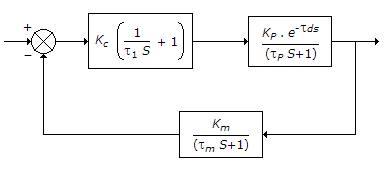# Chemical Engineering - Process Control and Instrumentation

### Exercise :: Process Control and Instrumentation - Section 3

36.

Measurement of sub-zero Celcius temperature in industry is done most commonly by

 A. thermocouples B. resistance thermometers C. gas thermometers D. bi-metallic thermometers

Explanation:

No answer description available for this question. Let us discuss.

37.

For the block diagram shown below :The characteristic equation is

 A. τl S (τps + 1) + Kc . Kp (τlS + 1) e-τd s = 0 B. (τmS + 1) (τpS + 1) + Km . Kp e-τd S = 0 C. τl S(τm S + 1) (τp S + 1) +Kc . Kp . Km (τl s + 1) e-τdS = 0 D. (τms + 1) (τp s + 1) + Kc . Kp . Km . e-τdS = 0

Explanation:

No answer description available for this question. Let us discuss.

38.

Use of I-control along with P-control facilitates

 A. elimination of offset B. reduction of offset C. reduction of stability time D. none of these

Explanation:

No answer description available for this question. Let us discuss.

39.

Turbine flow meters are suitable for

 A. very limited flow ranges. B. cryogenic flow measurements. C. aerospace and in air borne applications. D. both (b) and (c).

Explanation:

No answer description available for this question. Let us discuss.

40.

Liquid discharge from a tank or reservoir can not be measured by

 A. orificemeters B. weirs C. mouthpieces D. notches